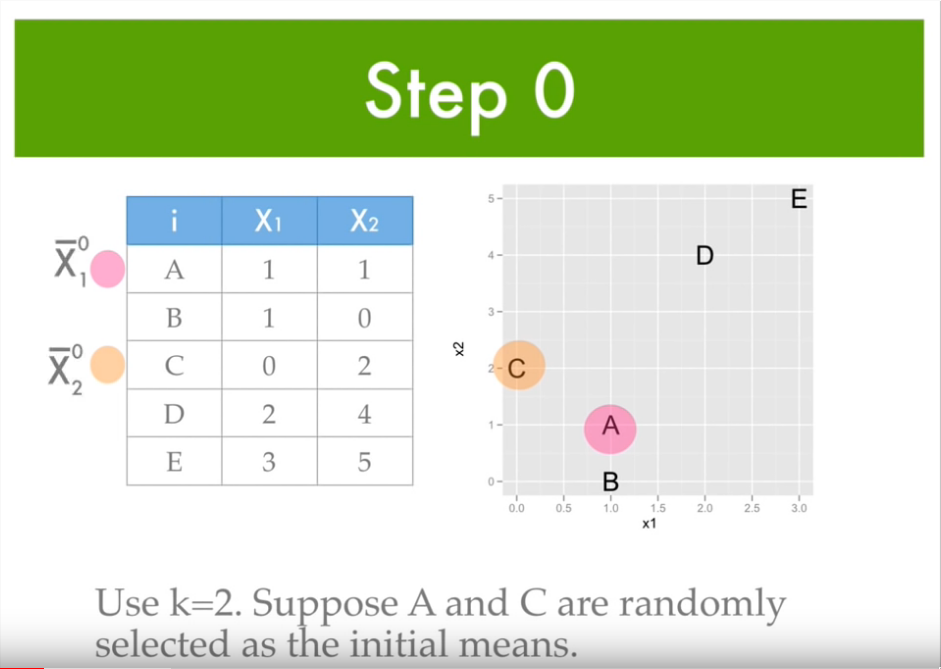1590780551

# K Means Clustering in pictures

Algorithm

1. Randomly pick K number of clusters and K number of centroids.

2. For each point, calculate the distance between centroids and place the point in the cluster whose centroid is closest.

3. After all points are assigned, find the centroids again (sum of x / total K , sum y / total K)

https://medium.com/analytics-vidhya/k-means-clustering-in-pictures-bc02da13fc08

#data-science #kmeans #machine-learning